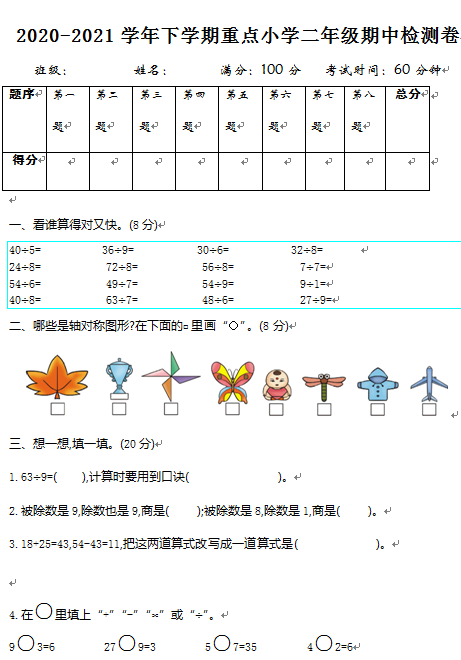2020-2021学年下学期重点小学二年级期中检测卷

40÷5=　　　　　36÷9=　　　　　30÷6=　　　　　32÷8=

24÷8=             72÷8=              56÷8=             7÷7=

54÷6=             49÷7=              54÷9=             9÷1=

40÷8=             63÷7=              48÷6=             27÷9=

【DOC文档6页】2020-2021学年下学期重点小学二年级数学期中检测卷(含答案)A4电子版资料_可直接打印_会员免费下载##### 评论信息